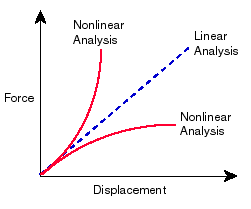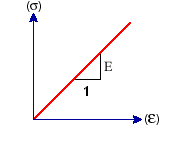# Linear Static Analysis

When loads are applied to a body, the body deforms and the effect of loads is transmitted throughout the body. The external loads induce internal forces and reactions to render the body into a state of equilibrium. Linear Static analysis calculates displacements, strains, stresses, and reaction forces under the effect of applied loads.

Linear static analysis makes the following assumptions:

Static Assumption

All loads are applied slowly and gradually until they reach their full magnitudes. After reaching their full magnitudes, loads remain constant (time-invariant). This assumption allows us to neglect inertial and damping forces due to negligibly small accelerations and velocities. Time-variant loads that induce considerable inertial and/or damping forces may warrant dynamic analysis. Dynamic loads change with time and in many cases induce considerable inertial and damping forces that cannot be neglected.

• It is important to verify the static assumption since a dynamic load may generate stresses up to 1/(2x) times the stresses generated by static loads with the same magnitude, where x is the viscous damping ratio. For a lightly damped structure with 5% damping, the dynamic stresses will be 10 times larger than the static stresses. The worst case scenario occurs at resonance. Please refer to the section of Dynamic Analysis.
• You can use static analysis to calculate the structural response of bodies spinning with constant velocities or traveling with constant accelerations since the generated loads do not change with time.
• Use linear or nonlinear dynamic studies to calculate the structural response due to dynamic loads. Dynamic loads include oscillatory loads, impacts, collisions, and random loads.

Linearity Assumption

The relationship between loads and induced responses is linear. For example, if you double the loads, the response of the model (displacements, strains, and stresses), will also double. You can make the linearity assumption if:

• All materials in the model comply with Hooke’s law, that is stress is directly proportional to strain.
• The induced displacements are small enough to ignore the change in stiffness caused by loading.
• Boundary conditions do not vary during the application of loads. Loads must be constant in magnitude, direction, and distribution. They should not change while the model is deforming.